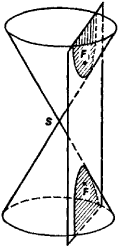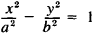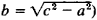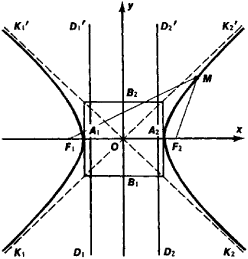# hyperbola

(redirected from hyperbolae)
Also found in: Dictionary, Thesaurus.
Related to hyperbolae: hyperbole

## hyperbola

(hīpûr`bələ), plane curve consisting of all points such that the difference between the distances from any point on the curve to two fixed points (foci) is the same for all points. It is the conic sectionconic section
or conic
, curve formed by the intersection of a plane and a right circular cone (conical surface). The ordinary conic sections are the circle, the ellipse, the parabola, and the hyperbola.
formed by a plane cutting both nappes of the conecone
or conical surface,
in mathematics, surface generated by a moving line (the generator) that passes through a given fixed point (the vertex) and continually intersects a given fixed curve (the directrix).
; it thus has two parts, or branches. The center of a hyperbola is the point halfway between its foci. The principal axis is the straight line through the foci. The vertices are the intersection of this axis with the curve. The transverse axis is the line segment joining the two vertices. The latus rectum is the chord through either focus perpendicular to the principal axis. The asymptotes are lines, in the same plane, which the curve approaches as it approaches infinity. An equilateral, or rectangular, hyperbola is one whose asymptotes are perpendicular. A second hyperbola may be drawn whose asymptotes are identical with those of the given hyperbola and whose principal axis is a perpendicular line through the center; the two hyperbolas thus related are called conjugate.

## hyperbola

(hÿ-per -bŏ-lă) A type of conic section that has an eccentricity greater than one. See also orbit.
Collins Dictionary of Astronomy © Market House Books Ltd, 2006

## Hyperbola

The section of a right circular cone by a plane that intersects the cone on both sides of the apex.
The following article is from The Great Soviet Encyclopedia (1979). It might be outdated or ideologically biased.

## Hyperbola

the curve of intersection of a circular cone with a plane cutting both of its nappes (Figure 1). A hyperbola may also be defined as the geometric locus of the points M in a plane, such that the difference of their distances from two fixed points F1 and F2 (foci of the hyperbola) in that plane is constant. If a coordinate system xOy is selected such asFigure 1

that represented in Figure 2 (OF1 = OF2 = c), then the equation of the hyperbola assumes the form(2a = F1M - F2M and b =. A hyperbola is a curve of the second order. Consisting of two infinite branches K1A1K1’ and K2A2K2’, it is symmetrical with respect to the F1F2 and B1B2 axes. The point O is the center of the hyperbola and the center of its symmetry. The segments A1A2 = 2a and B1B2= 2b are called, respectively, the transverse and conjugate axes of the hyperbola. The number e = c/a > 1 is the eccentricity of the hyperbola. The straight lines D1D1’ and D2D2’, whose equations are x = -a/e and x = a/e, are the directrixes of the hyperbola. The ratio of the distance of aFigure 2

point on the hyperbola from the nearest focus to the distance from the nearest directrix is constant and equal to the eccentricity. The points A1 and A2 of the hyperbola’s intersection with the Ox axis are called its vertices. The straight lines y = ± b/a (represented by dashed lines in Figure 2) are the asymptotes of the hyperbola. The graph of the inverse proportionality y = k/x is a hyperbola.

## hyperbola

[hī·pər·bə·lə]
(mathematics)
The plane curve obtained by intersecting a circular cone of two nappes with a plane parallel to the axis of the cone.
McGraw-Hill Dictionary of Scientific & Technical Terms, 6E, Copyright © 2003 by The McGraw-Hill Companies, Inc.

## hyperbola

a conic section formed by a plane that cuts both bases of a cone; it consists of two branches asymptotic to two intersecting fixed lines and has two foci. Standard equation: x2/a2 -- y2/b2 = 1 where 2a is the distance between the two intersections with the x-axis and b = a&#221A(e2 -- 1), where e is the eccentricity
Collins Discovery Encyclopedia, 1st edition © HarperCollins Publishers 2005
References in periodicals archive ?
The spherical hyperbolae include one of these foci and the opposite point in the other hemisphere, in each of two separate families of curves.
In areas of the sea where the sets of curves (for at least two pairs of transmitters) intersect reasonably transversal it is sufficient to measure two time differences, then a look on the sea chart shows the ships position as the intersection point of two spherical hyperbolae. Following Griffiths and Culpin (1975) we recall since the lines of equal sums of distances from two points on the sphere are related to the lines of equal absolute value of differences of distances it would seem likely that the ellipses as well as the hyperbolae should be divided into sub-families separated by a great circle.
Spherical ellipses and hyperbolae having the same foci intersect orthogonally.
The disadvantage of the graph in figure 2 is that constant cost curves are hyperbolae which are difficult to plot.
This is still a family of hyperbolae but the asymptote intersects the [t.sub.o](t) axis at [Delta], and, if [Delta] depends upon [t.sub.e], then each hyperbola in a family will have its own asymptote.
As regards the "inches" expression, it appears from Adagia 406 ("Latum vnguem, ac similes hyperbolae prouerbiales," ASD 2, 1) that Folly owes it to Plautus, Aulularia 56-57, "Si tu hercle ex isthoc loco digitum transuersum aut vnguem latum excesseris .

Site: Follow: Share:
Open / Close